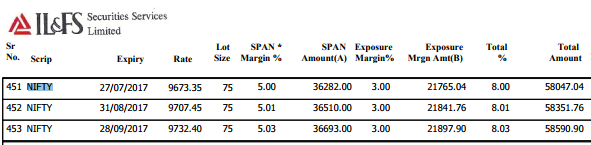# Highest intraday margin in FNO

Can anybody tell which broker provide highest intraday margin in FNO…
As per my knowledge fortune trading provide 20 times exposure in fno

1 Like

Hello @DA6704

Futures trading is already a margin product. You only require a Normal margin to carry forward an F&O trade and not the entire contract value.
When it comes to intraday trades, an MIS trade will require 40% of the Normal margin and a Bracket order trade requires only 40% of the MIS margin.

Let’s take an example with Nifty Futures:

Normal margin(NRML margin)
Nifty futures trades at 9670 and its lot size is 75. This makes the contract value Rs.7,25,250. The normal margin required to carry forward 1 lot of Nifty futures is 8% of the contract value( 5% span and 3% exposure) which is Rs.58,000.(12.5 times margin compared to contract value)Refer the normal margin list.

MIS Margin
An MIS trade for 1 lot of Nifty futures requires only 40% of normal margin. In this case it is 40% of 58k which is Rs.23,000. (32 times margin compared to contract value)

Refer the MIS margin calculator for Futures

Bracket order margin
A Bracket Order trade for 1 lot of Nifty futures requires only 40% of the MIS margin. In this case it is 40% of 23k which is Rs.9200. (80 times margin compared to contract value)

Refer the Bracket order calculator

Here is a tutorial on how to place a Bracket order on Youtube.

1 Like